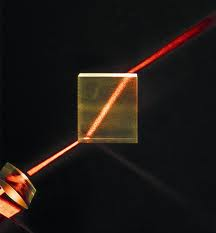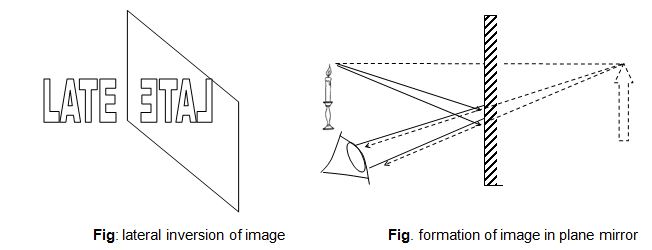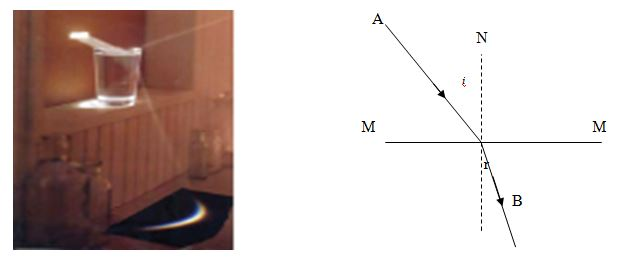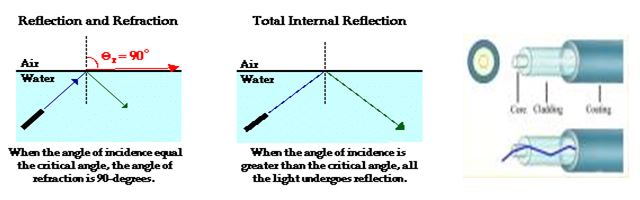Physics

# Assignment on Refraction of Light### Reflection of Light

The bouncing off of light or any wave at the face or boundary between two medium is called refraction.The figure above illustrates the regular reflection of a light ray. MM` represents the surface of a plane mirror. AO is the incident ray i.e. the direction in which light falls on to the reflecting surface. O is the point of incidence; OB is the reflected ray and ON is the normal on the reflecting surface. The angle of incidence i and the angle of reflection r are made by AO and OB respectively with the normal NO.### Laws of reflection

The laws of reflection are stated as follows:

Law (1): The incident and the reflected rays are on the opposite sides of the normal at the point of incidence and all three are in the same plane.

Law(2): The angle of incidence is equal to the angle of refraction.

### Image formation in a plane mirror

Light rays diverging out of the tip of a candle are reflected from the surface of the plane mirror MM`. Their extrapolations in back ward direction intersects at I. I is the position of the image of the tip of the candle. The eye sees the cone of rays E1IE2 seem to coming out of the Tip of the image I. however the AIB portion of the rays does not exist and hence the image is a virtual one.Another important property of the image formed by reflection is that it is laterally inverted, i.e. left becomes right and right, left. This is why    is written as    on the front hood of it. This is done for the drivers of the cars ahead of it who will see the ambulance in his rear view mirror and find the word in proper orientation-AMBULANCE.

### Refraction of light :

When light (or any wave) passes from one medium to another its direction changes at the boundary. This phenomenon of changing of direction at the boundary of two medium is called refraction. The angle of incidence is the angle made by the incident ray with  the normal  at the point of incidence. The angle of  refraction is the angle made by the refracted ray with the normal at the point of incidence.

When a ray passes from a medium to a more optically dense medium, the refracted ray bends toward the normal. Conversely, a ray passing from glass to water or water to air bends away  from the normal.### Laws of Refraction:

The laws of refraction are stated as follows:

Law (1): The incident and the refracted rays are on the opposite sides of the normal at the point of incidence and all three are in the same plane.

Law(2) (Snell’s law): The ratio of the sine of the angle of the incidence to the  sine of the angle of refraction is a constant for a given pair of media and a particular colour of light.

### Refractive index:

The value of the constant  for a ray passing from one medium to another is called the refractive index of the second medium with respect to the first; and is denoted .

If air is the first medium then n is simply referred to as the refractive index of the  second medium. In strict sense absolute refractive index is defined with respect to vacuum.

### Total internal reflection and critical angle:

When light passes from a medium to a less optically dense medium the ray moves away from the normal, i.e. the angle of refraction is greater than the angle of incidence. And this angle of refraction goes on increasing with the increase of angle of incidence in the denser medium. Finally at a certain critical angle of incidence c the angle of refraction becomes 900. At a greater angle of incidence light is totally internally reflected within the denser medium. This particular property of light enables it to be transmitted through a bent transparent fiber which is called optical fiber .